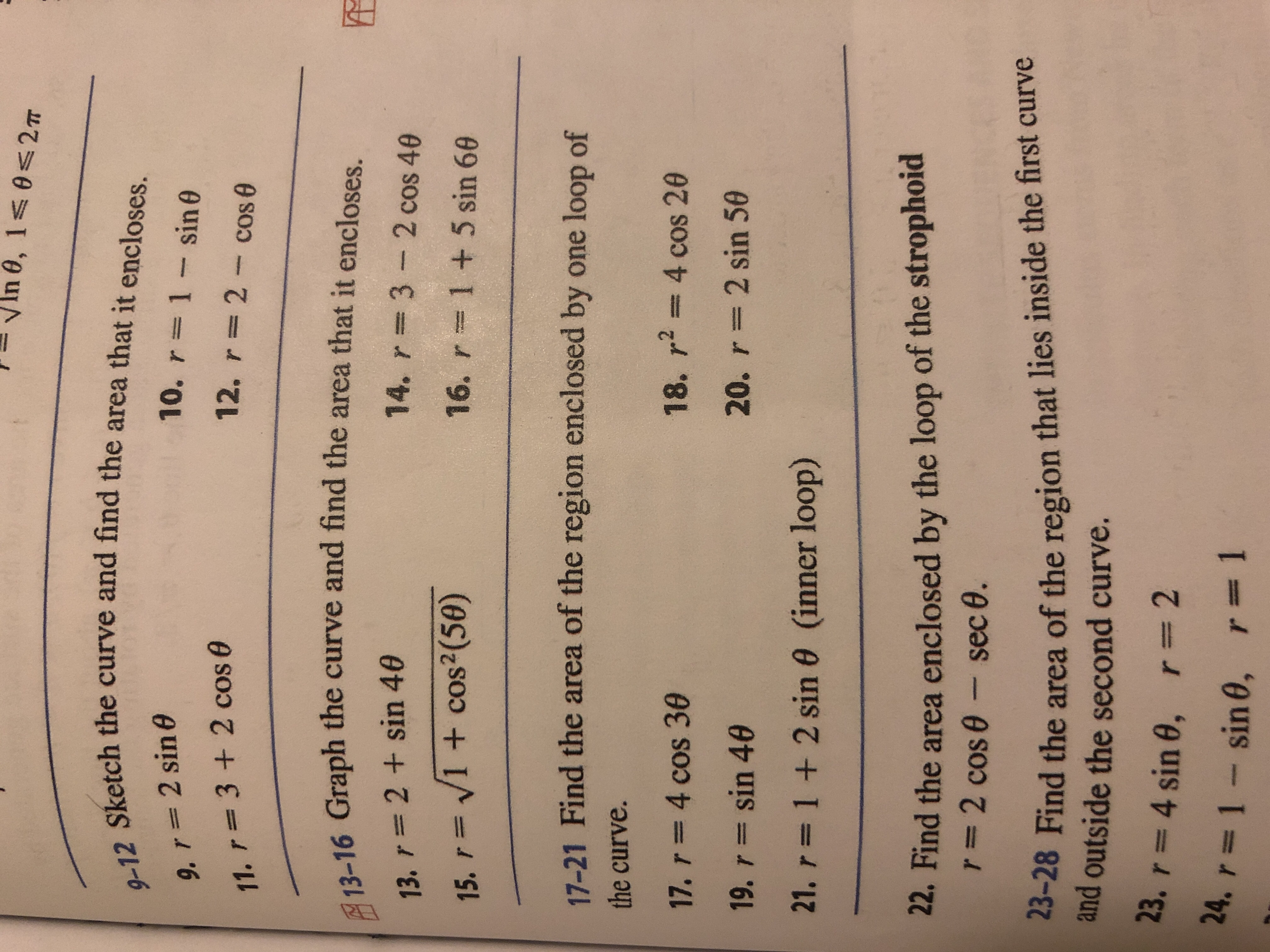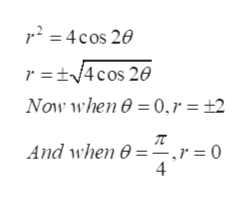# In 0, 1s 0 2T9-12 Sketch the curve and find the area that it encloses.10. r 1 sin 09. r 2 sin e12. r 2 cos 011. r3 +2 cos 013-16 Graph the curve and find the area that it encloses.14. r 3 2 cos 4013. r 2+sin 4016. r15. V1 + cos2 (50)1 + 5 sin 6017-21 Find the area of the region enclosed by one loop ofthe curve.18. r2 4 cos 2017. r 4 cos 3020. r 2 sin 5019. r sin 4021. r1+ 2 sin 0 (inner loop)22. Find the area enclosed by the loop of the strophoidr 2 cos e - sec 0.23-28 Find the area of the region that lies inside the first curveand outside the second curve.23. r 4 sin 0,r=224. r1sin 0, r= 1

Question
17 views

Find the area of the region enclosed by one loop of the curve.

18. r^2 = 4cos2(theta)help_outlineImage TranscriptioncloseIn 0, 1s 0 2T 9-12 Sketch the curve and find the area that it encloses. 10. r 1 sin 0 9. r 2 sin e 12. r 2 cos 0 11. r3 +2 cos 0 13-16 Graph the curve and find the area that it encloses. 14. r 3 2 cos 40 13. r 2+sin 40 16. r 15. V1 + cos2 (50) 1 + 5 sin 60 17-21 Find the area of the region enclosed by one loop of the curve. 18. r2 4 cos 20 17. r 4 cos 30 20. r 2 sin 50 19. r sin 40 21. r1+ 2 sin 0 (inner loop) 22. Find the area enclosed by the loop of the strophoid r 2 cos e - sec 0. 23-28 Find the area of the region that lies inside the first curve and outside the second curve. 23. r 4 sin 0, r=2 24. r1sin 0, r= 1 fullscreen
check_circle

Step 1

Refer to the question we need to find the area of the region enclosed by one loop of the curve.

Step 2

First graph the polar equation as ,help_outlineImage Transcriptionclosep2=4cos 20 r t4cos 20 Now when 0 0,r = 2 And when e=.r= 0 4 fullscreen
Step 3

Now sketch the graph as the graph will be s...

### Want to see the full answer?

See Solution

#### Want to see this answer and more?

Solutions are written by subject experts who are available 24/7. Questions are typically answered within 1 hour.*

See Solution
*Response times may vary by subject and question.
Tagged in

### Integration